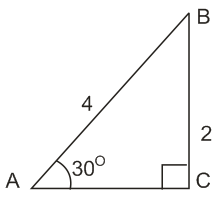# The angles A,B and C of a triangle ABC are in A.P.  and .If c = 4 cm , then the area ( in sq. cm) of this triangle is:  Option 1) Option 2) Option 3) Option 4)In  ,

A,B,C are in A.P.

=>

Now, it is given that a:b=

Now, Area of

Option 1)

Option 2)

Option 3)

Option 4)

Exams
Articles
Questions